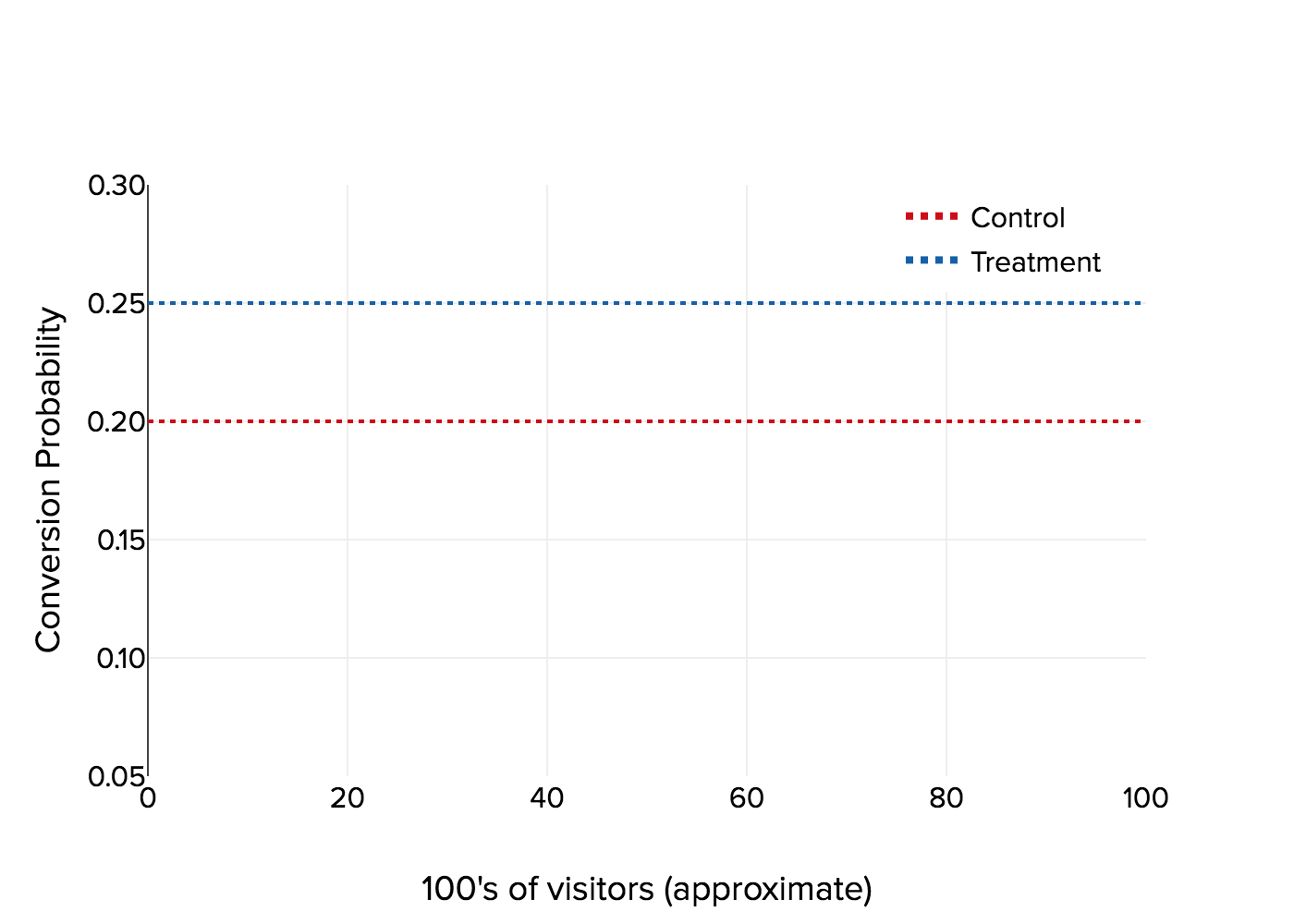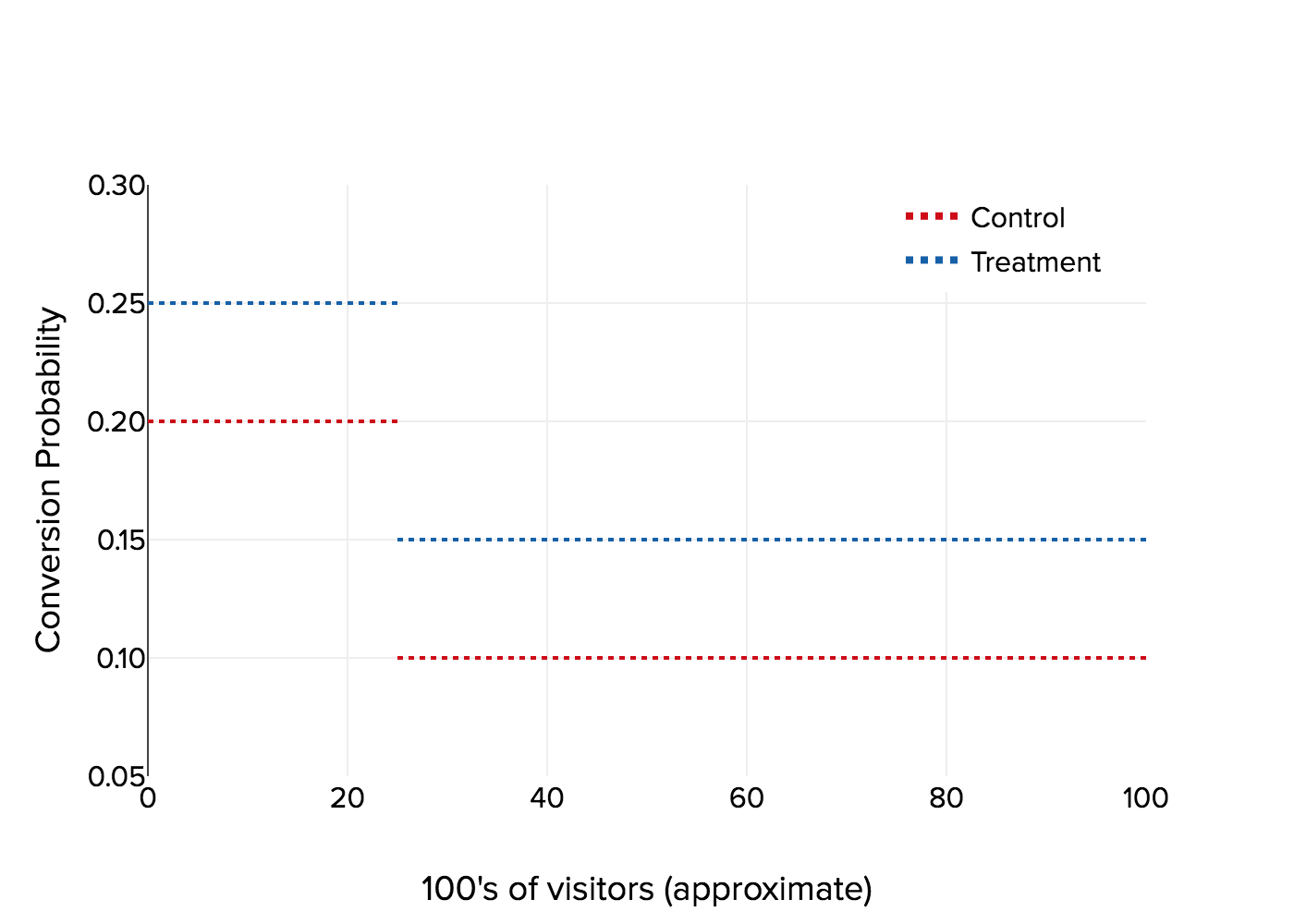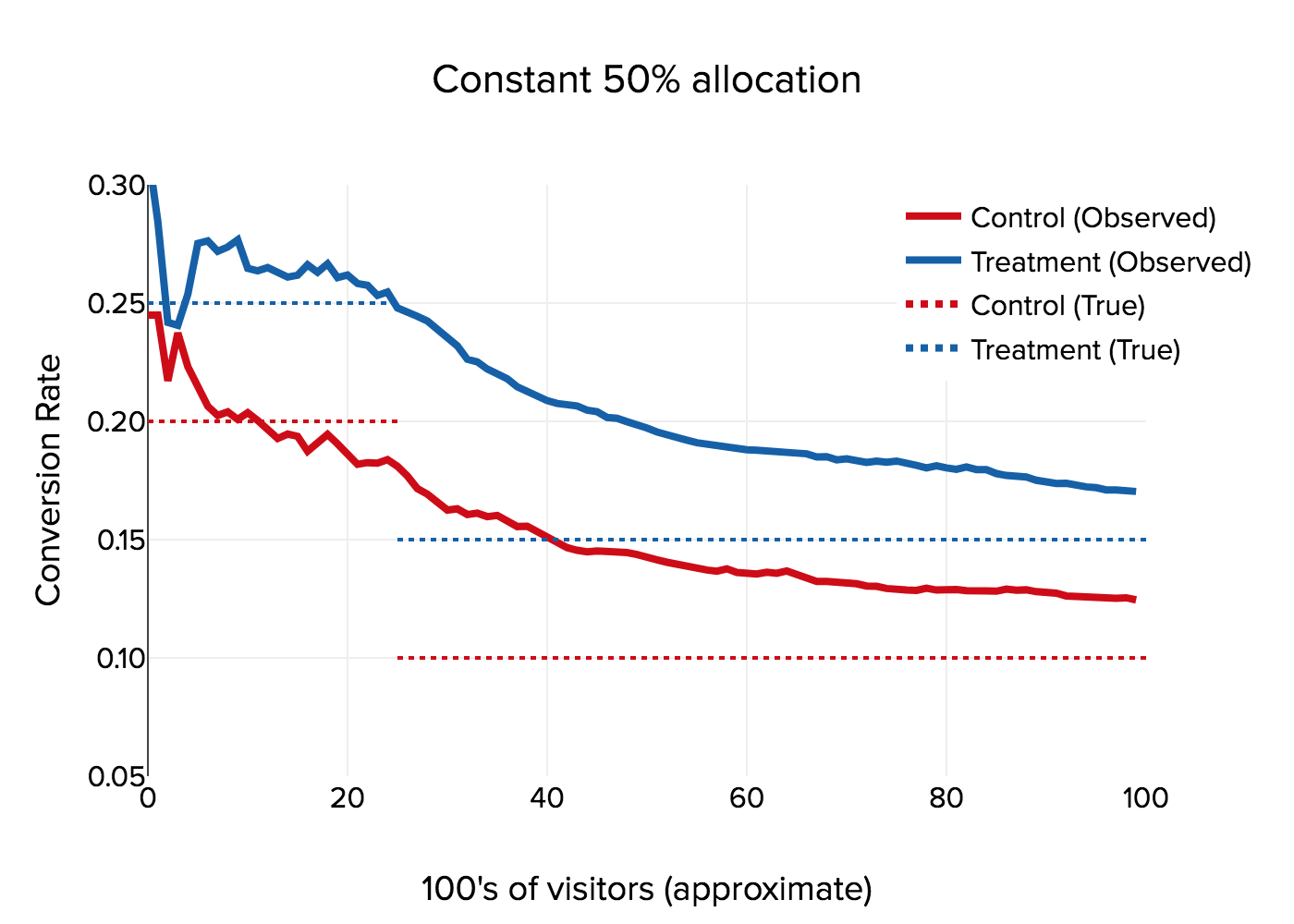# Stats Accelerator – Acceleration Under Time-Varying Signals

• Updated
This blog post was initially posted on Medium.com. It has been updated here to reflect any updates.

This is part two of a series on Stats Accelerator. We explained the when, why, and how of Stats Accelerator in the first part. Today’s installment will discuss a significant roadblock to the successful productionization of bandits in the A/B testing context and how we eventually overcame it. This is a high-level overview.

Back in 2017, Optimizely announced Stats Accelerator, a pair of multi-armed bandit algorithms for accelerating experimentation. These algorithms are designed to either optimize rewards for some time or identify a statistically significant variant as quickly as possible by intelligently changing the allocation of traffic between variations (or arms, in machine learning terminology) of the experiment.

However, when underlying conversion rates or means vary over time (e.g., due to seasonality), dynamic traffic allocation can cause substantial bias in estimates of the difference between the treatment and the control, a phenomenon known as Simpson’s Paradox. This bias can completely invalidate statistical results on experiments using Stats Accelerator, breaking usual guarantees on false discovery rate control.

To prevent this, we developed Epoch Stats Engine, a simple modification to our existing Stats Engine methodology, which makes it robust to Simpson’s Paradox. At its core is a stratified estimate of the difference in means between the control and the treatment. Because it requires no estimation of the underlying time variation and is compatible with other central limit theorem-based approaches such as the t-test, we believe it lifts a substantial roadblock to combining traditional hypothesis and bandit approaches to A/B testing in practice.

## What is time variation?

A fundamental assumption behind many A/B tests is that the underlying parameter values we are interested in do not change over time. When this assumption is violated, we say there is time variation. In the context of A/B experiments using Stats Accelerator, Simpson’s Paradox can only occur in the presence of time variation, so a precise understanding of time variation will be helpful in the future.

Let us take a step back. In each experiment, we imagine that there are underlying quantities that determine the performance of each variation; we are interested in measuring these quantities, but they cannot be observed. These are parameters. For example, in a conversion rate web experiment, we imagine that each page variation has some actual ability to induce a conversion for each visitor. If we express this ability in terms of the probability that each visitor will convert, then these true conversion probabilities for each variation would be the parameters of interest. Since parameters are unobserved, we must compute point estimates from the data to infer parameter values and decide whether the control or treatment is better. In the conversion rate example, the observed conversion rates would be point estimates for the true conversion probabilities of each variation.

Noise and randomness will cause point estimates to fluctuate. In the basic A/B scenario, we view these fluctuations as centered around a constant parameter value. However, when the parameter values themselves fluctuate over time, reflecting underlying changes in the actual performance of the control and treatment, we say that there is time variation.Figure 1a: The traditional, no time variation view of the parameter values behind an A/B test.Figure 1b: An example of time variation impacting underlying parameter values in an A/B test.

The classic example is seasonality. For example, it is often reasonable to suspect that visitors to a website during the workweek may behave differently than visitors on the weekends. Therefore, conversion rate experiments may see higher (or lower) observed conversion rates on weekdays compared to weekends, reflecting matching potential time variation in the true conversion rates, representing true underlying differences in how visitors behave on weekdays and weekends.

Time variation can also manifest as a one-time effect. A landing page with a banner for a 20%-off sale valid for December may generate a higher-than-usual number of purchases per visitor for December but then drop off after January arrives. This would be a time variation in the parameter representing the average number of purchases per visitor for that page.

Time variation can take different forms and affect your results in different ways. Whether time variation is cyclic (seasonality) or transient (a one-time effect), suggest other ways to interpret your results. Another critical distinction regards how the time variation affects different arms of the experiment. Symmetric time variation occurs when parameters vary across time so that all arms of the experiment are affected equally (in a sense to be defined shortly). Asymmetric time variation covers a broad swath of scenarios where this is not the case. Optimizely’s Stats Engine currently has a feature to detect substantial asymmetric time variation. It will reset your statistical results accordingly to avoid misleading conclusions, but handling asymmetric time variation generally requires solid assumptions and a wholly different type of analysis. This remains an open area of research.

In what follows, we will restrict ourselves to the symmetric case with an additive effect for simplicity. Specifically, we imagine the parameters for the control and treatment θC(t) and θT(t) may be written as θC(t) = μC + f(t) and θT(t) = μT + f(t) so that each can be decomposed into non-time-varying components μC and μT and the common source of the time variation f(t). The underlying lift may therefore be written by the non-time-dependent quantity μT – μC = θT(t) – θC(t).

Generally, the symmetric time variation occurs when the source of the time variation is not associated with a specific variation but rather the entire population of visitors. For example, visitors during the winter holiday season are more inclined to purchase in general. Therefore, a variation that induces more purchases will maintain the difference over the control even with a higher overall holiday-influenced click-through rate for both the variation and the control.

In general, most A/B testing procedures such as the t-test and sequential testing are robust to this type of time variation. This is because we are often less interested in estimating the individual parameters of the control and treatment and more interested in the difference between the parameters. Therefore, if both parameters are impacted in an additive manner by the same amount, then such time variation will be canceled out once differences are taken, and any subsequent inference will be relatively unaffected. Using the notation above, this can be seen in the fact that the difference in the time-varying parameters θT(t) – θC(t) = μT – μC does not contain the time-varying factor f(t).

As it turns out, though, the innocuous-seeming case of symmetric time variation can become a completely different beast when dynamic traffic allocation is introduced to the equation.

Suppose the traffic split in an experiment is adjusted in sync with the underlying time variation. A disproportionate amount of high- or low-performing traffic may be allocated to one arm relative to the other, biasing our view of the true difference between the two arms. This is a form of Simpson’s paradox, the phenomenon of a trend appearing in several different data groups that then disappear or reverse when the groups are aggregated together. This bias can completely invalidate experiments on any platform by tricking the stats methodology into declaring a larger or smaller effect size than what exists. Let’s motivate this by an example.

Consider a two-month conversion rate experiment with one control and one treatment. In November, the actual conversion rates for the control and treatment are at 10% and 20%, respectively. For December, they rise to 20% and 30%. The difference in conversion rates in each month is ten percentage points (pp).

Suppose traffic is split 50% to treatment and 50% to control (or any other proportion for that matter) for the entire duration of the experiment. In that case, the final estimate of the difference between the two variations should be close to 10%. What happens if traffic is split 50/50 in November but then changes to 75% to control and 25% for treatment in December? For simplicity, let’s assume that there are 1000 total visitors to the experiment each month. A simple calculation shows that:

Control:

Total visitors: 500 + 750 = 1250

Percent from high-converting regime: 750 / 1250 = 60%

Treatment:

Total visitors: 500 + 250 = 750

Percent from high-converting regime: 250 / 750 = 33%

So both control and treatment have equal numbers of visitors from low-converting November, but the treatment has far fewer visitors than the control from high-converting December. This imbalance clues us in that there will be bias, and doing the math confirms this: the conversion rate for the control will be around 16%, and the conversion rate for the treatment will be about 23%, a difference of only 7% rather than the 10% that we would typically expect.

This phenomenon is also laid clear in a continuous view, such as an Optimizely customer would witness:Figure 2a: Movement of observed conversion rates under time variation with constant traffic allocationFigure 2b: Movement of observed conversion rates under time variation with changing traffic allocation. The simulation initially begins at 50% allocation to treatment, switching to 90% allocation to treatment after 2,500 visitors.

In this example, the diminished point estimate might cause a statistical method to fail to report significance when it otherwise would with an unbiased point estimate closer to 10%.  But other adverse effects are also possible. When there is no true effect (e.g., we ran an A/A test), Simpson’s Paradox can cause the illusion of a significant positive or negative impact, leading to inflated false positives. Or when the time variation is extreme, or the traffic allocation syncs up well with the time variation. This bias can be so drastic as to reverse the sign of the estimated difference between the control and treatment parameters (as seen in Figure 2a), completely misleading experimenters as to the true effect of their proposed change.

## Epoch Stats Engine

Since Simpson’s Paradox manifests as a bias in the point estimate of the difference in means of the control and treatment, mitigating Simpson’s Paradox requires a way to remove such bias. In turn, eliminating bias requires accounting for one of the two factors causing it: time variation or dynamic traffic allocation. Since time variation is unknown and must be estimated, but traffic allocation is directly controlled by the customer or Optimizely and therefore known, we opted to focus on the latter.

Our solution for Simpson’s Paradox follows from the observation that bias due to Simpson’s paradox cannot occur over periods of constant traffic allocation. Therefore, we may derive an unbiased point estimate by making estimates within periods of constant allocation (called epochs) and then aggregating those individual within-epoch estimates to obtain one unbiased across-epoch estimate. Suppose we can show that this quantity is compatible with the sequential testing methodology underneath Stats Engine’s hood. In that case, we have a plug-and-play solution that works seamlessly with experiments using Stats Accelerator. As we will also see, this estimator is also simple enough to be applied to other statistical tests such as the t-test.

Let us get into the math a bit. Suppose there are K(n) total epochs when the experiment has seen n visitors. Within each epoch k, denote by nk,C and nk,T the sample sizes of the control and treatment respectively, and by k and k the sample means of the control and treatment respectively. Letting nk = nk,C + nk,T, the epoch estimator for the difference in means isBecause the dependence across epochs induced by the data-dependent allocation rule is restricted to changes in the relative balance of traffic between the control and treatment, the within-epoch estimates are orthogonal, and the variance for Tn is well-estimated by the sum of the estimated variances of each within-epoch component:Where σ̂C and σ̂T are consistent estimates for the standard deviations of the data-generating processes for the control and treatment arms.

Tn is a stratified estimate at a high-level n is a stratified estimate where the strata represent data belonging to individual epochs of fixed allocation. At a low level, this is a weighted estimate of within-epoch estimates. The weight assigned to each epoch is proportional to the total number of visitors within that epoch. We also surface the epoch estimate as “Weighted improvement” on the results page for experiments using Stats Accelerator.Figure 3: Calculation of an epoch stratified estimate (computed at 15,000 visitors) of the difference in true conversion probabilities in an experiment with a traffic allocation change and one-time symmetric time variation, both occurring at 10,000 visitors.

It is worth repeating that the epoch estimate is guaranteed to be unbiased since each within-epoch estimate is unbiased. In addition, we provide rigorous guarantees that the epoch estimate is fully compatible with the sequential test employed at Optimizely and generally valid for use in other central limit theorem-based methods (such as the t-test). See the full write-up for more details.

## Performance on simulated data

We simulated data with time variation and ran four different Stats Engine configurations on that data:

1. Standard  Stats Engine
2. Epoch Stats Engine
3. Standard Stats Engine with Accelerate Learnings
4. Epoch Stats Engine with Accelerate Learnings

Specifically, we generated 600,000 draws from 7 Bernoulli arms with one control and six variants, one truly higher-converting arm and all others converting at the same rate as the control. The conversion rate for the control starts at 0.10 and then undergoes cyclic time variation rising as high as 0.15. In each of these plots, we plot visitors on the horizontal axis and either false discovery rate (FDR) or true discovery rate (TDR) on the vertical axis, averaging over 1000 simulations.Figure 4: Average false discovery rate over time for the simulation scenario described above.

The FDR plot shows that Epoch Stats Engine does exactly what we designed it to do–protect customers from false discoveries due to Simpson’s Paradox. The non-epoch bandit policy’s FDR exceeds the configured FDR level (0.10) by up to 150%. In contrast, the epoch-enabled bandit policy shows proper control of FDR at levels comparable to those achieved by Stats Engine without the bandit policy enabled. The main goal is to bring FDR levels of bandit-enhanced A/B testing down to levels similar to no bandit enabled.Figure 5: Average true discovery rate over time for the simulation scenario described above.

The TDR plot shows that we do not lose much power due to switching from standard to Epoch Stats Engine. First, we observe a large gap between the bandit allocation runs and the fixed allocation runs, reflecting that speedup due to bandit allocation is preserved under Epoch Stats Engine. Furthermore, we observe little difference in time to significance between the epoch and non-epoch scenarios under fixed allocation. In contrast, we observe a small gap in time to significance between the epoch and non-epoch scenarios under the bandit policy. This gap can be ascribed to the fact that the non-epoch Stats Engine runs under dynamic allocation and experiences high sensitivity to time variation, especially after crossing an epoch boundary, thereby creating the scalloped shape of the blue curve. Higher TPR is paid for with higher FDR.

## Conclusion

At Optimizely, we pride ourselves on pushing the envelope in A/B testing while always prioritizing statistical rigor to ensure customers make the most impactful decisions.

As we saw in the previous blog post, the latest iteration on this theme produced Stats Accelerator, a marriage of multi-armed bandit techniques with false discovery rate control. In Epoch Stats Engine, we’ve surmounted a major real-world obstacle to safe productionizing of this technology and have developed a solution that is:

1. proven to work well with sequential testing in both theory and simulation
2. simple to compute and understand, and
3. widely applicable to other methods and platforms, not just sequential testing and Optimizely

Are you interested in a deeper dive? Check out the technical paper!

<% if (previousArticle || nextArticle) { %>
<% if (previousArticle) { %>
<% if (previousTitle) { %>

#### <%= previousTitle %>

<% } %> <%= previousArticle.title %>
<% } %> <% if (nextArticle) { %>
<% if (nextTitle) { %>

#### <%= nextTitle %>

<% } %> <%= nextArticle.title %>
<% } %>
<% } %>
<% if (allItems.length > 1) { %>